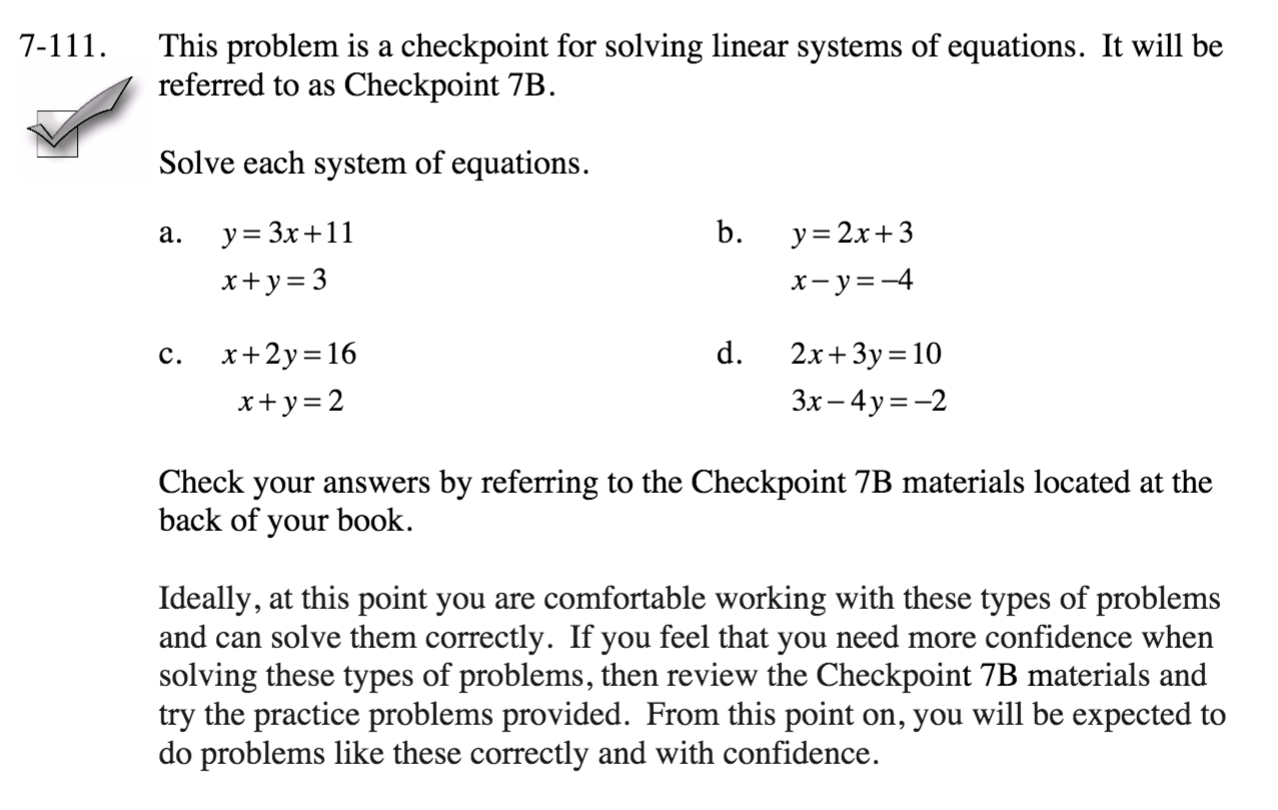### Home > CCA > Chapter 7 > Lesson 7.2.3 > Problem7-111

7-111.
1.This problem is a checkpoint for solving linear systems of equations. It will be referred to as Checkpoint 7B.

2. Solve each system of equations. Homework Help ✎

3.  a. y = 3x + 11 x + y = 3 b. y = 2x + 3 x – y = –4 c. x + 2y = 16 x + y = 2 d. 2x + 3y = 10 3x – 4y = –2
4. Check your answers by referring to the Checkpoint 7B materials.

5. Ideally, at this point you are comfortable working with these types of problems and can solve them correctly. If you feel that you need more confidence when solving these types of problems, then review the Checkpoint 7B materials and try the practice problems provided. From this point on, you will be expected to do problems like these correctly and with confidence.Answers and extra practice are located in the back of your printed textbook or in the Reference Tab of your eBook.
If you have an eBook for CCA, login and then click the following link: Checkpoint 7B: Solving Linear Systems of Equations